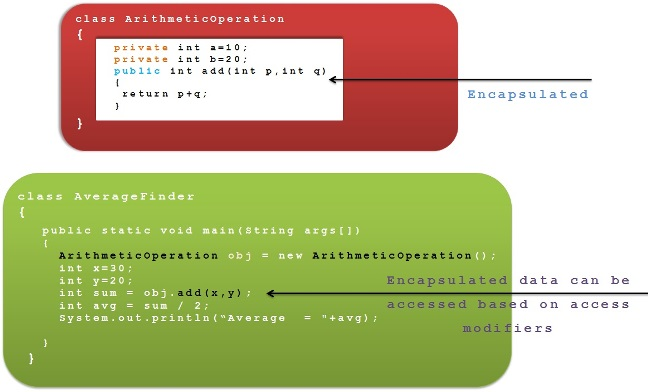# Encapsulation

##### Encapsulation

Process of binding object state(fields) and behaviors(methods) together in a single entity called “Class”.

Encapsulation wraps both fields and methods in a class, It will be secured from the outside access.We can restrict the access to the members of a class using access modifiers along with encapsulation.

When we create a class in Java, It means we are doing encapsulation.

Encapsulation helps us to achieve the reusability of code without compromising the security.

###### Example

We create a class and write a method to add 2 numbers.

Now , Addition logic can be accessed by other class if they also need to add 2 numbers rather than writing the whole logic again.

In this scenario , we can keep fields of our class as `private `and make add() method as `public`

So that other classes can access only the logic and can’t access the `private `fields and hence our data will be secured.

This way they are able to reuse the existing Add numbers logic.###### Let’s see the example program for the same

ArithmeticOperation.java

1. Class ArithmeticOperation{
2. Private Int a=10;
3. Private Int b=20;
4. Public int add(int p,int q){
5. return p+q;
6. }
7. }
```Class ArithmeticOperation{
Private Int a=10;
Private Int b=20;
return p+q;
}
}```

AverageFinder.java

1. Class  AverageFinder{
2.
3. public static void main(String args[]){
4. ArithmeticOperation obj = new ArithmeticOperation();
5. int x=30;
6. int y=20;
8. int avg = sum / 2;
9. System.out.println(“Average  = "+avg);
10.
11. }
12.
13. }
```Class  AverageFinder{

public static void main(String args[]){
ArithmeticOperation obj = new ArithmeticOperation();
int x=30;
int y=20;
int avg = sum / 2;
System.out.println(“Average  = "+avg);

}

}```

We can see that , AverageFinder class is reusing the addition logic encapsulated inside ArithmeticOperation class.

The fields declared inside ArithmeticOperation(both a and b) are secured as we declared those fields as `private`.